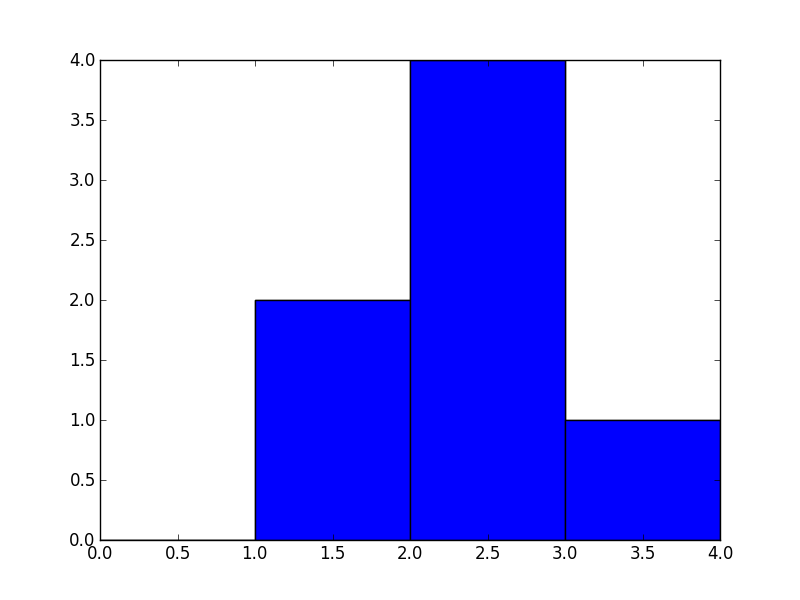# Chapter 18: Modular Programming¶

### Example 18.2, Page number: 364¶

In :
# Variable declaration
array = [1, 2, 3, 4, 5]
array_size = 10
num = 6

# Calculation
for index in range (5, 10) :
array.insert(index, num)
num = num + 1

# Result
print "Contents of array of size 10 elements is", array

Contents of array of size 10 elements is [1, 2, 3, 4, 5, 6, 7, 8, 9, 10]


### Example 18.3, Page number: 372¶

In :
# Calculation
import numpy as np

# hist indicates that there are 0 items in bin #0, 2 in bin #1, 4 in bin #3, 1 in bin #4
# bin_edges indicates that bin #0 is the interval [0,1), bin #1 is [1,2), ..., bin #3 is [3,4)

hist, bin_edges = np.histogram([1, 1, 2, 2, 2, 2, 3], bins = range(5))

# Result
import matplotlib.pyplot as plt
plt.bar(bin_edges[:-1], hist, width=1) and plt.xlim(min(bin_edges), max(bin_edges))
plt.savefig('histogram.png')

from IPython.core.display import Image
Image(filename='histogram.png')

Out: